Gain-Scheduled Implicit and Explicit MPC Control of Mass-Spring System

This example shows how to use an Multiple MPC Controllers block and an Multiple Explicit MPC Controllers block to implement gain scheduled MPC control of a nonlinear plant.

System Description

The system is composed by two masses M1 and M2 connected to two springs k1 and k2 respectively. The collision is assumed completely inelastic. Mass M1 is pulled by a force F, which is the manipulated variable. The objective is to make mass M1's position y1 track a given reference r.

The dynamics are twofold: when the masses are detached, M1 moves freely. Otherwise, M1 and M2 move together. We assume that only M1 position and a contact sensor are available for feedback. The latter is used to trigger switching the MPC controllers. Note that the position and velocity of mass M2 are not controllable.

/-----\     k1                 ||
F <--- | M1  |----/\/\/\-------------[|| wall
||                   | |---/                        ||
||     k2            \-/      /----\                ||
wall||]--/\/\/\-------------------| M2 |                ||
||                            \----/                ||
||                                                  ||
----yeq2------------------ y1 ------ y2 ----------------yeq1----> y axis

The model is a simplified version of the model proposed in .

Model Parameters

M1 = 1;       % mass
M2 = 5;       % mass
k1 = 1;       % spring constant
k2 = 0.1;     % spring constant
b1 = 0.3;     % friction coefficient
b2 = 0.8;     % friction coefficient
yeq1 = 10;    % wall mount position
yeq2 = -10;   % wall mount position

Plant Models

The state-space plant models for this examples have the following input and output signals:

• States: Position and velocity of mass M1

• Manipulated variable: Pulling force F

• Measured disturbance: Constant value of 1 which calibrates the spring force to the correct value

• Measured output: Position of mass M1

Define the state-space model of M1 when the masses are not in contact.

A1 = [0 1;-k1/M1 -b1/M1];
B1 = [0 0;-1/M1 k1*yeq1/M1];
C1 = [1 0];
D1 = [0 0];
sys1 = ss(A1,B1,C1,D1);
sys1 = setmpcsignals(sys1,'MD',2);
-->Assuming unspecified input signals are manipulated variables.

Define the state-space model when the two masses are in contact.

A2 = [0 1;-(k1+k2)/(M1+M2) -(b1+b2)/(M1+M2)];
B2 = [0 0;-1/(M1+M2) (k1*yeq1+k2*yeq2)/(M1+M2)];
C2 = [1 0];
D2 = [0 0];
sys2 = ss(A2,B2,C2,D2);
sys2 = setmpcsignals(sys2,'MD',2);
-->Assuming unspecified input signals are manipulated variables.

Design MPC Controllers

Specify the controller sample time Ts, prediction horizon p, and control horizon m.

Ts = 0.2;
p = 20;
m = 1;

Design the first MPC controller for the case when mass M1 detaches from M2.

MPC1 = mpc(sys1,Ts,p,m);
MPC1.Weights.OV = 1;
-->The "Weights.ManipulatedVariables" property of "mpc" object is empty. Assuming default 0.00000.
-->The "Weights.ManipulatedVariablesRate" property of "mpc" object is empty. Assuming default 0.10000.
-->The "Weights.OutputVariables" property of "mpc" object is empty. Assuming default 1.00000.

Specify constraints on the manipulated variable.

MPC1.MV = struct('Min',0,'Max',30,'RateMin',-10,'RateMax',10);

Design the second MPC controller for the case when masses M1 and M2 are together.

MPC2 = mpc(sys2,Ts,p,m);
MPC2.Weights.OV = 1;
-->The "Weights.ManipulatedVariables" property of "mpc" object is empty. Assuming default 0.00000.
-->The "Weights.ManipulatedVariablesRate" property of "mpc" object is empty. Assuming default 0.10000.
-->The "Weights.OutputVariables" property of "mpc" object is empty. Assuming default 1.00000.

Specify constraints on the manipulated variable.

MPC2.MV = struct('Min',0,'Max',30,'RateMin',-10,'RateMax',10);

Simulate Gain Scheduled MPC in Simulink®

Simulate gain-scheduled MPC control using the Multiple MPC Controllers block, which switches between MPC1 and MPC2.

% To run this example, Simulink(R) is required.
disp('Simulink is required to run this example.')
return
end

Open and configure the model.

y1initial = 0;      % Initial position of M1
y2initial = 10;     % Initial position of M2
mdl = 'mpc_switching';
open_system(mdl)
if exist('animationmpc_switchoff','var') && animationmpc_switchoff
close_system([mdl '/Animation'])
clear animationmpc_switchoff
end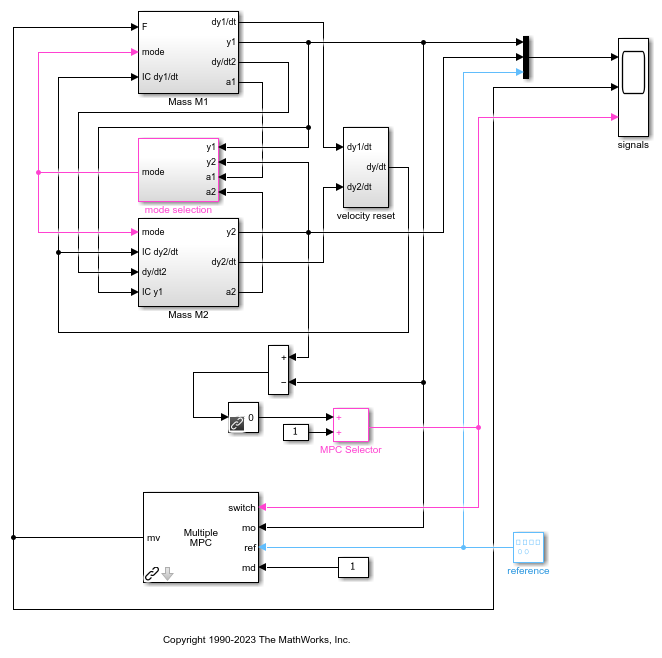Simulate the model.

open_system([mdl '/signals'])
sim(mdl)
MPC1saved = MPC1;
MPC2saved = MPC2;
-->Converting model to discrete time.
-->Assuming output disturbance added to measured output channel #1 is integrated white noise.
-->The "Model.Noise" property of the "mpc" object is empty. Assuming white noise on each measured output channel.
-->Converting model to discrete time.
-->Assuming output disturbance added to measured output channel #1 is integrated white noise.
-->The "Model.Noise" property of the "mpc" object is empty. Assuming white noise on each measured output channel.
-->Converting model to discrete time.
-->Assuming output disturbance added to measured output channel #1 is integrated white noise.
-->The "Model.Noise" property of the "mpc" object is empty. Assuming white noise on each measured output channel.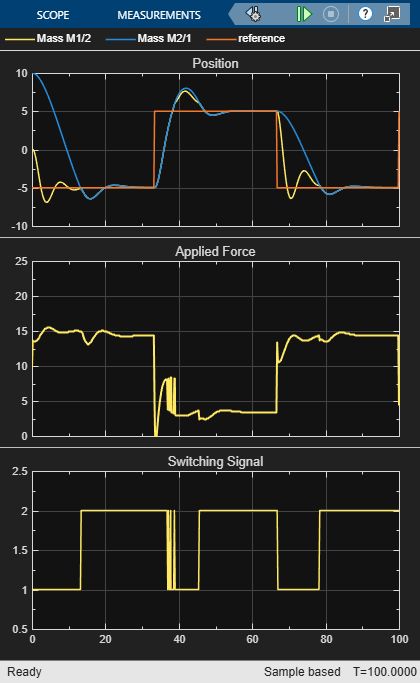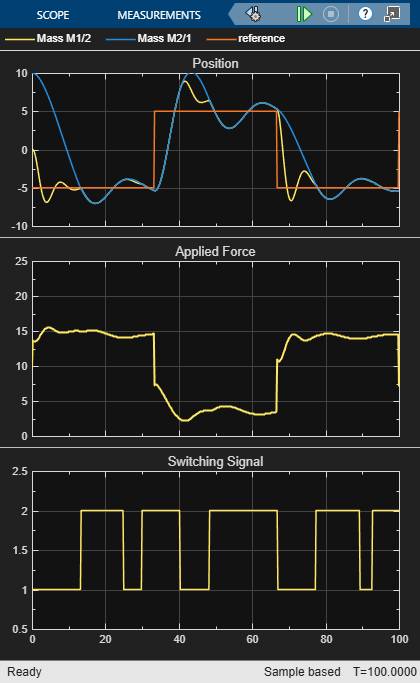Using two controllers provides satisfactory performance under all conditions.

Repeat Simulation Using MPC1 Only

Repeat the simulation assuming that the masses are never in contact; that is, using only controller MPC1.

MPC1 = MPC1saved;
MPC2 = MPC1saved;
sim(mdl)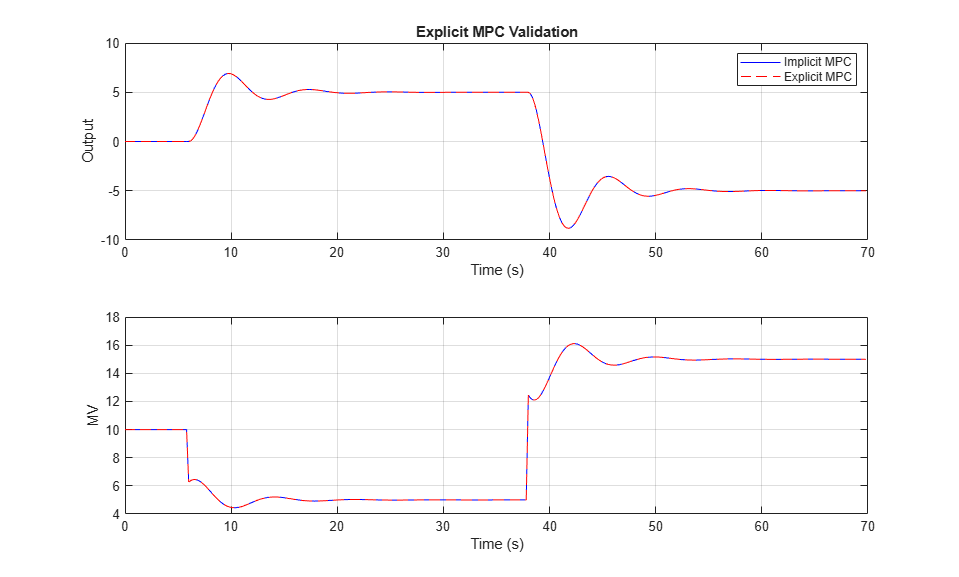In this case, performance degrades whenever the two masses join.

Repeat Simulation Using MPC2 Only

Repeat the simulation assuming that the masses are always in contact; that is, using only controller MPC2.

MPC1 = MPC2saved;
MPC2 = MPC2saved;
sim(mdl)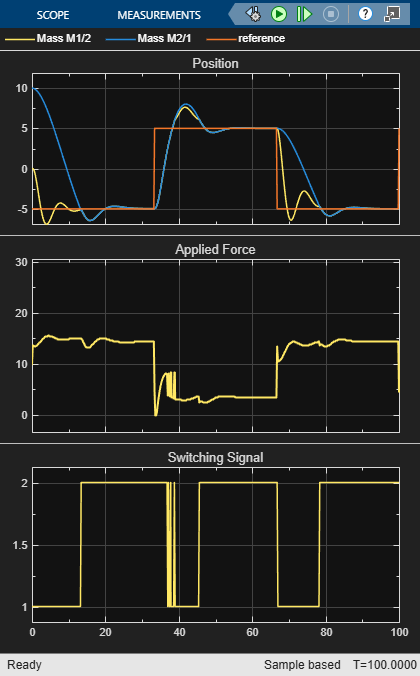In this case, performance degrades when the masses separate, causing the controller to apply excessive force.

bdclose(mdl);
close(findobj('Tag','mpc_switching_demo'));

Design Explicit MPC Controllers

To reduce online computational effort, you can create an explicit MPC controller for each operating condition, and implement gain-scheduled explicit MPC control using the Multiple Explicit MPC Controllers block.

To create an explicit MPC controller, first define the operating ranges for the controller states, input signals, and reference signals. Create an explicit MPC range object using the corresponding traditional controller, MPC1.

range = generateExplicitRange(MPC1saved);

Specify the ranges for the controller states. Both MPC1 and MPC2 contain states for:

• The position and velocity of mass M1

• An integrator from the default output disturbance model

range.State.Min(:) = [-10;-8;-3];
range.State.Max(:) = [10;8;3];

When possible, use your knowledge of the plant to define the state ranges. However, it can be difficult when the state does not correspond to a physical parameter, such as for the output disturbance model state. In that case, collect range information using simulations with typical reference and disturbance signals. For this system, you can activate the optional est.state outport of the Multiple MPC Controllers block, and view the estimated states using a scope. When simulating the controller responses, use a reference signal that covers the expected operating range.

Define the range for the reference signal. Select a reference range that is smaller than the M1 position range.

range.Reference.Min = -8;
range.Reference.Max = 8;

Specify the manipulated variable range using the defined MV constraints.

range.ManipulatedVariable.Min = 0;
range.ManipulatedVariable.Max = 30;

Define the range for the measured disturbance signal. Since the measured disturbance is constant, specify a small range around the constant value, 1.

range.MeasuredDisturbance.Min = 0.9;
range.MeasuredDisturbance.Max = 1.1;

Create an explicit MPC controller that corresponds to MPC1 using the specified range object.

expMPC1 = generateExplicitMPC(MPC1saved,range);

Regions found / unexplored:        4/       0

Create an explicit MPC controller that corresponds to MPC2. Since MPC1 and MPC2 operate over the same state and input ranges, and have the same constraints, you can use the same range object.

expMPC2 = generateExplicitMPC(MPC2saved,range);

Regions found / unexplored:        5/       0

In general, the explicit MPC ranges of different controllers may not match. For example, the controllers may have different constraints or state ranges. In such cases, create a separate explicit MPC range object for each controller.

Validate Explicit MPC Controllers

It is good practice to validate the performance of each explicit MPC controller before implementing gain-scheduled explicit MPC. For example, to compare the performance of MPC1 and expMPC1, simulate the closed-loop response of each controller using sim.

r = [zeros(30,1); 5*ones(160,1); -5*ones(160,1)];
[Yimp,Timp,Uimp] = sim(MPC1saved,350,r,1);
[Yexp,Texp,Uexp] = sim(expMPC1,350,r,1);
-->Converting model to discrete time.
-->Assuming output disturbance added to measured output channel #1 is integrated white noise.
-->The "Model.Noise" property of the "mpc" object is empty. Assuming white noise on each measured output channel.

Compare the plant output and manipulated variable sequences for the two controllers.

figure
subplot(2,1,1)
plot(Timp,Yimp,'b-',Texp,Yexp,'r--')
grid on
xlabel('Time (s)')
ylabel('Output')
title('Explicit MPC Validation')
legend('Implicit MPC','Explicit MPC')
subplot(2,1,2)
plot(Timp,Uimp,'b-',Texp,Uexp,'r--')
grid on
ylabel('MV')
xlabel('Time (s)')The closed-loop responses and manipulated variable sequences of the implicit and explicit controllers match. Similarly, you can validate the performance of expMPC2 against that of MPC2.

If the responses of the implicit and explicit controllers do not match, adjust the explicit MPC ranges, and create a new explicit MPC controller.

Simulate Gain-Scheduled Explicit MPC

To implement gain-scheduled explicit MPC control, replace the Multiple MPC Controllers block with the Multiple Explicit MPC Controllers block.

expModel = 'mpc_switching_explicit';
open_system(expModel)Run the simulation.

sim(expModel)To view the simulation results, open the signals scope.

open_system([expModel '/signals'])The gain-scheduled explicit MPC controllers provide the same performance as the gain-scheduled implicit MPC controllers.

References

 A. Bemporad, S. Di Cairano, I. V. Kolmanovsky, and D. Hrovat, "Hybrid modeling and control of a multibody magnetic actuator for automotive applications," in Proc. 46th IEEE® Conf. on Decision and Control, New Orleans, LA, 2007.

bdclose(expModel)
close(findobj('Tag','mpc_switching_demo'))